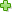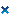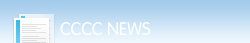报名热线：0551-65690888 65690999首 页 职院首页 职院新闻 职院简介 教育教学 专业设置 就业保障 视频教学 校园生活 在线报名 联系我们首页 >职业教育学院 >教育教学 >正文
Excel表中在身份证号码里提取出生年月、性别、年龄的使用技巧
 来源：教研室   编辑：赵凯   日期：12-01-21

方法一:

1.Excel表中用身份证号码中取其中的号码用:MID(文本,开始字符,所取字符数);
2.15位身份证号从第7位到第12位是出生年月日，年份用的是2位数。
18位身份证号从第7位到第14位是出生的年月日，年份用的是4位数。
从身份证号码中提取出表示出生年、月、日的数字，用文本函数MID()可以达到目的。MID()――从指定位置开始提取指定个数的字符（从左向右）。
对一个身份证号码是15位或是18位进行判断，用逻辑判断函数IF()和字符个数计算函数LEN()辅助使用可以完成。综合上述分析，可以通过下述操作，完成形如1978-   12-24样式的出生年月日自动提取：

如果只要“年-月”格式，公式可以修改为=IF(LEN(A1)=15,MID(A1,7,2)&"-"&MID(A1,9,2),MID(A1,7,4)&"-"&MID(A1,11,2))
3.这是根据身份证号码（15位和18位通用）自动提取性别的自编公式，供需要的朋友参考：
说明：公式中的B2是身份证号
根据身份证号码求性别：
=IF(LEN(B2)=15,IF(MOD(VALUE(RIGHT(B2,3)),2)=0,"女","男"),IF(LEN(B2)=18,IF(MOD(VALUE(MID(B2,15,1)),2)=0,"女","男"),"身份证错"))
根据身份证号码求年龄：
=IF(LEN(B2)=15,2007-VALUE(MID(B2,7,2)),if(LEN(B2)=18,2007-VALUE(MID(B2,7,4)),"身份证错"))
4.Excel表中用Year\Month\Day函数取相应的年月日数据;

这是根据身份证号码（15位和18位通用）自动提取性别和出生年月的自编公式，供需要的网友参考：
说明：公式中的B2是身份证号

1.根据身份证号码求性别：
=IF(LEN(B2)=15,IF(MOD(VALUE(RIGHT(B2,3)),2)=0,"女","男"),IF(LEN(B2)=18,IF(MOD(VALUE(MID(B2,15,3)),2)=0,"女","男"),"身份证错"))
2.根据身份证号码求出生年月：
=IF(LEN(B2)=15,CONCATENATE("19",MID(B2,7,2),".",MID(B2,9,2)),IF(LEN(B2)=18,CONCATENATE(MID(B2,7,4),".",MID(B2,11,2)),"身份证错"))
3.根据身份证号码求年

9 7 3 1 2 4 8 :关闭窗口最新新闻教育部原副部长张天保出席我院淘宝职院新校区建设项目7月即将破土动互惠多赢，校企合作新发展我院新校区列入省政府重点协调调度就业安置新亮点：分类就业好“出口视觉冲击：人的包装新学问“我是新生”系列四：打开新生活的“我是新生”系列三：学成归去报恩2010级新生开班典礼：新起点上我院学生应邀参加“迎四体会倒计时“我是新生”系列二：不可阻挡的潮